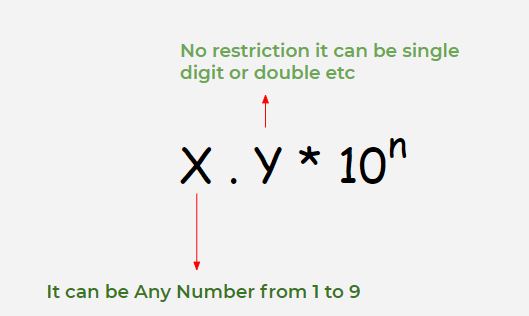Open in App
Not now

# Laws of Exponents & Use of Exponents to Express Small Numbers in Standard Form – Exponents and Powers | Class 8 Maths

• Last Updated : 09 Jun, 2022

It is known that numbers can be expressed as xn where ‘x’ is called “Base” and ‘n’ is called as “Exponent”. In simple words, we can say that the Significance of the exponent is that it tells the number of times we need to multiply our base. There are certain Laws of exponents which will make the calculation easier and faster. Let’s see Laws along with examples, in all the examples we have taken x as 5 for better understanding, x can be any number.

## Let’s Discuss each of the laws in more detail

### Law 1

If we have any number as base and exponent of that base is 0 then answer will be 1 .

For Example:

20 = 1

30 =1

120 = 1

### Law 2

If we have any number as base and exponent of that base is 1 the answer is base itself.

For Example:

71 = 7

211 = 21

151 = 15

### Law 3

If we have any number as base and exponent of that base is -1 then  answer will be reciprocal of that base.

For Example:

8-1 = 1 / 8

15-1 = 1 / 15

27-1 = 1 / 27

### Law 4

If  we have to multiply two numbers with same base and different exponents then

xaxb = xa + b

x3x = (x * x * x) * (x * x * x * x)

= (x * x * x * x * x * x * x)

= x(3 + 4)

= x7

For Example:

2423  = 2(4 + 3)

= 27

7574 = 7(5 + 4)

= 79

(12)6(12)2 = 12(6 + 2)

= 128

### Law 5

If we have to divide two numbers with same base and different exponents then

xa / xb  = xa – b

x5 / x3  = (x * x * x * x * x) / (x * x * x)

= (x * x)= x(5 – 3)

= x2

For Example:

34 / 3 =  3(4 – 2)

= 32

58 / 53   =  5(8 – 3)

=  55

(13)7 / (13)5 = 13(7 – 5)

= 132

### Law 6

(xa)b  =  xab

(x2) 3 =  (x * x)3

= (x * x) (x * x) (x * x)

= (x * x * x * x * x * x) =  x(2 * 3)

= (x)6

For Example:

(23)4 = 2(3 * 4)

= 212

(52)3 = 5(2 * 3)

= 56

(134)5 = (13)(4 * 5)

= 1320

### Law 7

If we have two numbers to multiply with different base but same exponent then

(x * y)a = xaya

(x * y)4 = (xy) (xy) (xy) (xy)

= xyxyxyxy

= xxxxyyyy = x4y4

For Example:

(5 * 4)2 = 52 * 42

(7 * 3)4 = 74 * 34

(12 * 32)9 = 129  * 329

### Law 8

If we have to divide two numbers with different base but same exponent then

(x / y)a = xa / y a

(x / y)3  = (x/y)(x/y)(x/y)

= (x * x * x) / (y * y * y)

= x / y3

For Example:

(2 / 3)4 = 24 / 34

(6 / 8)2 = 6 / 82

(15 / 27)8 = 15 / 278

x-a = 1 / xa

For Example:

8 -2 = 1 / 8-2

7-3 = 1 / 73

15-6 = 1 / 156

## Use of Exponents to Express Small Number in Standard Form

### What is a Standard Form of Number ??Many times it Happens that we encounter a number that is very small to read and write properly, so for that purpose, there’s a better way of Describing those small numbers in Standard form.

Examples:

1. Diameter of a computer chip is 0.000003m = 3 * 10-6m
2. Mass of dust particle is 0.000000000753 kg = 7.53 * 10-10 kg
3. The length of the shortest visible wavelength of visible light (violet) is 0.0000004 m. = 4.0 * 10-7 m

These numbers are very small so we will convert them to standard form lets see the steps:

• Step I: Move the Decimal to the right until there’s only 1(non-zero) Digit to the left of the decimal.
• Step II: Suppose we have shifted the decimal by n place to right then multiply the remaining number by 10-n.

Examples:

• 0.000000000753 =  7.53 * 10-10
• 0.0000004           =  4 * 10-7
• 0.0000000894     =  8.94 * 10-8
• 0.00000000052   =  5.2 * 10-10
My Personal Notes arrow_drop_up
Related Articles